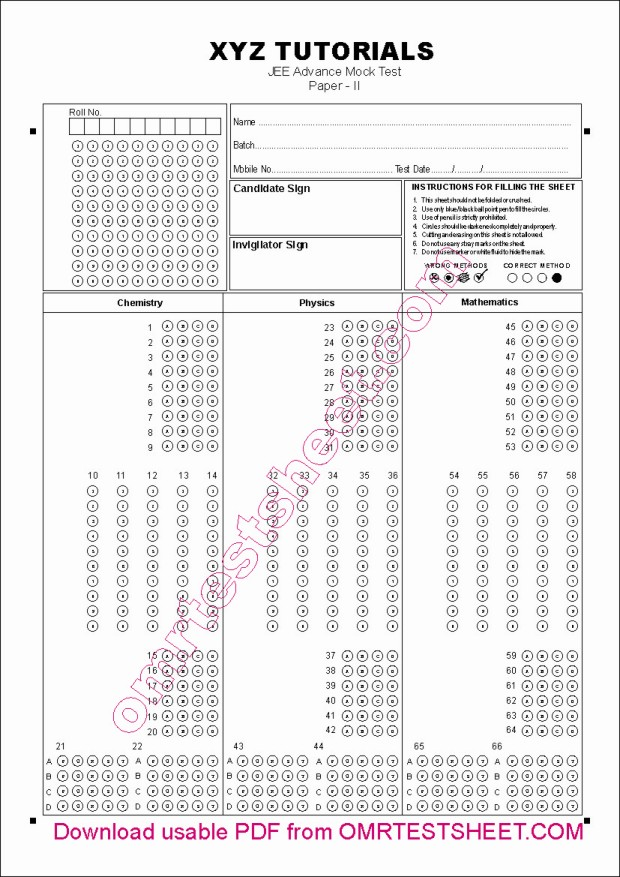# OMR Design with MCQ Block

Simple ABCD type; Numerical (Integer) Type; Matrix (Column matching) type

In this IIT JEE OMR Sheet for Practice with 66 Questions, there are 3 sections for Physics, Chemistry & Math’s each section having 22 questions. Question nos. 1-9 is single choice MCQs i.e. questions having ABCD type of MCQ OMR block. Question nos.10-14 is SINGLE DIGIT INTEGER type of questions with 1 column for single digit. Question nos. 15-20 is MCQ multiple choice questions having ABCD type of MCQ OMR block. Question nos. 21-22 is a Matrix matching type of questions with 4 columns (P, Q, R, and S) and 4 rows (A, B, and C, D).

For Example, Correct Answer to Q 20: A=P; B=R; C=S; D=Q; The answers for this type of Matrix blocks are entered in the following manner: P-R-S-Q